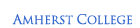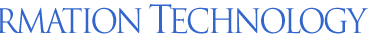ATS > Software > Scientific Programming with Python > Simulating a Magnet# Scientific Programming with Python

## Part 3: Simulating a Magnet

#### Current: Simulating a Magnet

NumPy provides a number of tools useful for simulations and for working with simple data types.

## The Ising Model

The Ising model is a simple model of a ferromagnet, using a regular lattice of magnetic “spins” that influence each other to line up, fighting against entropy all the while.

### Magnetic Spins

Magnets are atomic lattices with unpaired electrons, producing a spin magnetic moment at each position of the lattice:

↑ ↑ ↑ ↑ ↑ ↑ ↑ ↑ ↑ ↑          ↑ ↑ ↑ ↑ ↑ ↓ ↓ ↑ ↑ ↑          ↑ → ← ↑ ↑ ↓ ← ↑ → →

↑ ↑ ↑ ↑ ↑ ↑ ↓ ↑ ↑ ↑          ↑ ↑ ↑ ↑ ↑ ↓ ↓ ↓ ↑ ↑          ↓ ← ↑ ↓ ← → ↓ ← → ↑

↑ ↑ ↑ ↑ ↑ ↑ ↑ ↑ ↑ ↑          ↑ ↑ ← ← ↑ ↑ ↑ ↑ ↑ ↑          ← ↓ → ↓ ← ↑ ← ↑ ← ↓

↑ ↑ ↑ ← ↑ ↑ ↑ ↑ ↑ ↑          ↑ ← ← ← ↑ ↑ → → → →          ↓ ← → ← ↑ ← ↓ → ↓ →

↑ ↑ ↑ ↑ ↑ ↑ ↑ ↑ ↑ ↑          ↑ ↑ ↑ ↑ ↑ ↑ → → → ↑          ← ↑ ↓ ↓ → ↑ ← → ↑ ↓

Low Temperature             Medium Temperature            High TemperatureThese spins are tiny magnets and like to align with each other, and collectively can add up to a macroscopically measurable magnetization, such as in the bar magnet at the right:The higher the temperature, however, the more the thermal energy randomizes the spins, and the uniform alignment is broken up by patches of spins in other directions called domains, as in the red and green regions in the micrograph at the left.

Above a critical temperature there is no longer a dominant magnetic direction, and the domain magnetizations cancel each other out.

At infinite temperature the spins are completely random in their orientation even over short distances.

We can describe the tendency of the spins to line up in terms of the (potential) energy of the spin interaction. Over the entire lattice of size N it is given by

E = Σij Jij si·sj

where Jij is a positive coupling constant that incorporates the magnetic moment. Then,

• an alignment of the spins will produce a positive dot product and lower the energy, which is a more favorable state;
• an anti-alignment of the spins will produce a negative dot product and increase the energy, which is a less favorable state.

Complete spin alignment will have a large negative energy, while thermally randomized spins will have a higher energy near zero.

To simplify the model we can:

• establish a single z axis so that the spins in the vector dot product si·sj can be replaced by their z-component si = ±1 ;
• limit the range of the interaction to only nearest-neighbor pairs, which can be written into the sum and allowJ to be a single value.

The energy thereby becomes

E = J Σnn sisj

And we can represent the atomic lattice by an array of ±1 values:

  1 -1 -1  1  1 -1  1  1  1 -1
-1 -1 -1  1 -1 -1 -1  1  1  1
1 -1  1  1 -1  1  1 -1 -1  1
-1 -1  1 -1  1  1 -1 -1  1  1
-1 -1 -1  1 -1  1  1  1  1 -1
1 -1 -1  1 -1  1  1  1  1  1
1 -1 -1 -1  1 -1 -1 -1 -1 -1
-1 -1  1 -1 -1 -1  1 -1 -1 -1
-1 -1  1  1 -1 -1 -1 -1 -1  1
-1 -1  1  1 -1 -1 -1  1 -1  1

This is known as the Ising Model of magnets, and as we will see it retains their basic characteristics.

When the spins substantially align, there will be a measureable magnetization:

M = (1/N)∣Σ si

• When T → 0, the spins completely align, and E → –2NJ and M → 1.
• When T → ∞, the spins will be randomly oriented, and E → 0 and M → 0.

The magnetization is an example of an order parameter, which we can use to monitor the system order.

### Spyns

Not surprisingly, we can implement an Ising system and calculate its magnetization using Python.

We’ll use a two-dimensional lattice of size N = L × L:

L = 10
N = L**2

NumPy can define arrays with preset values in a number of ways. For example, to create a system at zero temperature, all spins aligned, we use:

import numpy as np
spins = np.ones((L,L), int)
print(spins)

⇒
[[1 1 1 1 1 1 1 1 1 1]
[1 1 1 1 1 1 1 1 1 1]
[1 1 1 1 1 1 1 1 1 1]
[1 1 1 1 1 1 1 1 1 1]
[1 1 1 1 1 1 1 1 1 1]
[1 1 1 1 1 1 1 1 1 1]
[1 1 1 1 1 1 1 1 1 1]
[1 1 1 1 1 1 1 1 1 1]
[1 1 1 1 1 1 1 1 1 1]
[1 1 1 1 1 1 1 1 1 1]]

The first argument to ones() is the size of the lattice, as an n-tuple for an n-dimensional lattice.

The second argument sets the data type, in this case NumPy integers (otherwise it would produce a float).

The magnetization will tell us the degree of order in the model:

M = lambda : np.absolute(np.sum(spins))/N
print('M = ', M())

⇒ 1.0

Perfectly ordered, by design!

Warning: in Python 2.x, you’ll want to use float(N) in the above expression to avoid integer truncation.

The function np.sum() simply adds together all elements of the array it’s handed as an argument.

To create a system at infinite temperature, with a random distribution of values, we can use a subset of NumPy tools, numpy.random, that provides random number generators:

import numpy.random as npr
spins = npr.randint(0, 2, (L,L)) * 2 - 1
print(spins)

[[ 1  1  1  1  1 -1 -1 -1 -1  1]
[ 1 -1  1  1 -1  1 -1 -1  1 -1]
[ 1 -1 -1  1  1 -1  1  1  1  1]
[-1  1  1  1  1  1 -1 -1 -1 -1]
[-1 -1 -1  1 -1 -1 -1  1  1 -1]
[-1  1  1 -1 -1 -1  1  1 -1  1]
[ 1  1 -1 -1  1  1  1 -1  1  1]
[-1  1  1 -1  1 -1  1  1 -1 -1]
[ 1 -1  1  1 -1 -1  1 -1  1 -1]
[-1 -1  1  1 -1  1 -1  1  1 -1]]

The first two arguments to randint() provide the range of integer values, minimum (inclusive) to maximum (exclusive), here producing values in the range (0, 1).

The third argument to randint() is the size of the lattice, again as an n-tuple for an n-dimensional lattice, here (L, L).

The result is a NumPy array of integers (0, 1), which allows broadcast calculations, here multiplication by 2 and then subtraction of 1 to produce the desired values of (–1, 1).

The magnetization is close to zero, as it should be for a completely disordered state:

print('M = ', M())

⇒ M = 0.06

It will never be precisely zero because it’s defined here, essentially, as the standard deviation of the average spin, but the larger the lattice we choose, the closer to zero it will be:

M = (1/N)∣Σ si~ (1/NN1/2 ~ 1/N1/2

So for N = 100, M ~ 0.1, as seen in the code snippet above.

### Spyn Statistics

For a given temperature, how can we put this system in an equilibrium state so that we can calculate its order parameter? First we better define what we mean by “equilibrium”.

Statistical mechanics tells us that, for a system in equilibrium at a temperature T, representing a bath of thermal energy that randomizes the spins, the probability of a particular configuration of the spins is proportional to its Boltzmann factor:

P({si}) ∝ exp(–E/kT)

where the Boltzmann constant k “converts” temperature to a corresponding energy.

Clearly the lowest energy state will have the highest probability.

For the Ising Model:

P({si}) ∝ exp((–J Σnn sisj)/kT)
= exp(β Σnn sisj )

where β = J/kT varies between ∞ and 0 as the temperature T varies between 0 and ∞.

In particular,

• When T → ∞ and β → 0, the exponential is equal to 1 for every pair of spins no matter what their orientation, meaning all states are equally likely, and there will be just as many “up” spins as “down” spins, on average.
• When T → 0 and β → ∞, the exponential is largest when the spins are all +1 or all –1, i.e. they all line up in one of those two directions.

Now consider an individual spin si and its neighbors sinn; it has an energy relative to its neighbors of

Ei = –(J /2) si Σnnsinn

where the factor of 1/2 avoids double-counting the energy once we include the neighboring spins. It’s helpful to define a spin sum Si as:

Si = siΣnnsinn

Ei = –(J /2)Si

The probability of a particular microstate si given neighbors sinn is then:

P(si|sinn) ∝ exp(–Ei/kT)
= exp((β/2)Si)

Distinct examples of the individual spin sum, energy, and probability are:

 Configuration 1  1 –1  1     1 -1 -1  1 -1    -1 1 -1  1 -1    -1 1 1  1 -1   -1 1 1  1 -1    1 1  1  1  1     1 –1 –1 –1 –1    –1 Si = –4 -4 -2 0 +2 +4 +4 Ei = +2J +2J +J 0 –J –2J –2J P(si|sinn)∝ exp(-2β) < 1 exp(-2β) < 1 exp(-β) < 1 1 exp(+β) > 1 exp(+2β) > 1 exp(+2β) > 1

We can again see that:

• when the spins are less aligned, Si < 0 and Ei > 0 and P(si|sinn) < 1;
• when the spins are more aligned Si > 0 and Ei < 0 and P(si|sinn) > 1.

In addition, note that flipping the central spin will always invert the signs of Si and Ei.

Now suppose we were to flip the central spin and this lowered the energy, e.g. we went from the second (blue) configuration in the table above to the last (pink) configuration. The spin sum is higher and the probability is also higher.

On the other hand, if flipping the central spin increased the energy, e.g. going from the last (pink) configuration to the second (blue) configuration, the spin sum will be lower and the probabilty will also be lower.

When in equilibrium the spins will flip back and forth over time, driven by the random energy of the temperature bath. However, on average, the system retains its state, in particular its degree of order. This means that the probability P of a microstate times the rate of transition W out of that microstate must be the same as the reverse process, i.e. over time the transition probabilities are equal:

P(si|sinn)W(si → –si) = P(–si|sinn)W(–sisi)

This condition is known as detailed balance, and is what we mean when we say a system is in equilibrium.

Detailed balance can also be used to drive a nonequilbrium system to equilibrium by flipping spins in a compatible manner, and this is the key to simulating such a system.

The ratio of the two transition rates is given by

W(–sisi)/ W(si → –si) = P(si|sinn)/P(–si|sinn)
= exp((β/2)Si) / exp(–(β/2)Si)
= exp(βSi)

Suppose flipping the spin si → –si lowers the energy; again this means that initially Ei > 0 and Si < 0. Because only the above ratio is important, we can choose this transition rate equal to 1, i.e. the spin will always flip:

W(Ehigh → Elow) = 1

Consequently, when flipping the spin si → –si increases the energy, initially Ei < 0 and Si > 0, we must have

W(Elow → Ehigh) = exp(–βSi) < 1

and the spin only has a fractional probability of flipping.

Increasing the energy is therefore less likely than decreasing it, and after repeated flipping attempts the lower energy state will become the most probable state, so that a nonequilbrium system must approach equilibrium over time.

## Monte Carlo Simulations

Because of the randomizing effect of thermal energy and the probabilistic nature of the system energetics, we can use random numbers to equilibrate a system.

### The Monte Carlo Procedure

Suppose we start with a system of spins at infinite temperature, which means they are randomly either ±1. We then instantaneously lower the temperature, a process known as quenching, and allow the system to equilibrate, or anneal.

Alternatively, for low temperatures, we can start with a system at zero temperature, with a uniform distribution of either +1 or –1, and then instantantaneously raise the temperature and allow it to equilibrate.

We can simulate such systems as follows:

1. Generate a lattice with the known initial configuration;
2. Randomly pick a spin;
3. Calculate its spin sum Si = siΣnnsinn;
4. If Si is less than or equal to zero, we flip it, since W(Ehigh → Elow) = 1;
5. Otherwise, calculate W(Elow → Ehigh) = exp(–βSi);
1. Calculate a uniformly distributed random number 0 ≤ R < 1 and compare it to W;
2. If R < W, flip the spin (because a small transition rate W means a low probability of flipping);

This Monte Carlo procedure is a standard practice in statistical science as a way to calculate the properties of complex systems. It is sometimes called Markov-Chain Monte Carlo (MCMC), since each state in the calculation depends only the immediately previous state.

It is also often referred to as the Metropolis Algorithm, after the first author of the 1953 paper Equation of State Calculations by Fast Computing Machines (who were listed in alphabetical order). However, the second author, computational physicist Arianna Rosenbluth, is credited with the actual implementation of the algorithm on one of the room-sized computers of the time, the MANIAC I at Los Alamos Scientific Laboratory in New Mexico:### The Monte Python Simulation

We’ll save the previous code in a file named ising.py!

import numpy as np
import
numpy.random as npr
L = 10
N = L**2
M = lambda : np.absolute(np.sum(spins))/N
spins = npr.randint(0, 2, (L,L)) * 2 - 1
# More code here.
print(spins)
print('M = ', M())

We’ll also set a relatively large value of β that should produce some amount of ordering since it corresponds to a low temperature:

beta = 2

Now the next steps will take place in a loop after generating the spins, starting by randomly picking an initial spin, as sequential picking of spins would generate spatial correlations:

i = 0
while i < N :
i += 1
s = tuple(npr.randint(0, L, 2))

This last line returns two values in the range (0, L–1) as a NumPy array. It is converted to a tuple s=(sx, sy), indices into the array spins.

 1 -1 -1 sl  s sr  1 sb  1 -1
-1 -1 -1  1 sb -1 -1  1  1  1
1 -1  1  1 -1  1  1 -1 -1  1
-1 -1  1 -1  1  1 -1 -1  1 st
sr -1 -1  1  1 st  1  1 sl  s
1 -1 -1 -1 sl  s sr  1  1 sb
st -1 -1  1 -1 sb -1 -1 -1 -1
s sr  1 -1 -1 -1  1 -1 -1 sl
sb -1  1  1 -1 -1 -1 st -1  1
-1 -1  1  1 st -1 sl  s sr  1


Now locate the indices of the adjacent spins, left, right, bottom, and top:

(sx, sy) = s
sl = (sx-1, sy)
sr = ((sx+1)%L, sy)
sb = (sx, sy-1)
st = (sx, (sy+1)%L)

When the spin s is at the left or bottom, one index is 0, and (0-1)-1. But Python defines slices so that negative indices count backward from the largest value, i.e. the index -1 is equivalent to the index L-1, again wrapping around to the right or top edge.

On the other hand, when the spin s is on the right or top edge, one index is L-1, so that (L-1+1)L, which Python treats as out-of-bounds. But we can use the modulus operator %, which provides the remainder when dividing two integers, to always produce a value within the range (0, L–1), specifically (L-1+1)%L0, wrapping around to the left edge or bottom edge.

These are known as circular boundary conditions and they help to reduce errors associated with finite lattice size.

Now calculate the spin sum Si = siΣnnsinn:

S = spins[s] * ( spins[sl] + spins[sr] + spins[sb] + spins[st] )

Finally test for the energy condition:

if S <= 0 :
spins[s] *= -1
else:
W = np.exp(-beta*S)
R = npr.rand()
if R < W : spins[s] *= -1

There is another random number generator here, rand(), which returns a floating point number between 0 (inclusive) and 1 (exclusive).

Once i reaches N, this loop terminates. We can calculate the matrix and magnetization at this time to see how they have changed, with the following statements that are not indented so they are only calculated once:

print(spins)
print('M = ', M())

Passing through the lattice once, on average, represents a single unit of time, since the entire lattice should have the chance to update once, no matter what its size. To run the loop for multiple units of time, define a number of time steps tmax and multiply it by the lattice size:

tmax = 100
i = 0
while
i < tmax * N :
i += 1
s = tuple(npr.randint(0, L, 2))
sl = (sx-1,sy)
sr = ((sx+1)%L,sy)
sb = (sx,sy-1)
st = (sx,(sy+1)%L)
S = spins[s] * ( spins[sl] + spins[sr] + spins[sb] + spins[st] )
if S <= 0 :
spins[s] *= -1
else:
W = np.exp(-beta*S)
R = npr.rand()
if R < W : spins[s] *= -1

### Ensembles

The calculations above are for particular lattice sizes, and over particular periods of time. But they are also for particular configurations of spins, which have a probability

P({si}) ∝ Πiexp(–Ei/kT) = exp(ΣiEi/kT) = exp(–E/kT)

To calculate the order parameter M, we would use a weighted average over all configuration of the spins, known as an ensemble:

M⟩ = Σi PiMi

Since a large lattice will have a huge number of configurations, 2N, it’s impractical to calculate every one.

However, a random selection of spins such as above can be repeated multiple times, and that should sample the configuration space. Each of the selected configurations will occur with its own relative probabilty, and after Z samples the average result should provide a good approximation, known as the sample mean:

M⟩ = (1/(Z−1))ΣZ M

(The factor of Z–1 occurs due to a loss of one degree of freedom in a sample.) We can therefore add a loop to the above spin sample that repeats, for example, Z = 100 times, and accumulate each of the “measured” magnetizations to calculate this mean.

We can also calculate the sample mean of the magnetization squared:

M2⟩ = (1/(Z−1))ΣZ M 2

which allows the calculation of the sample variance of M around the mean, given by:

sM2 = (1/(Z−1))ΣZ(M − ⟨M)2
= (1/(Z−1))ΣZ(M 2 − 2M ⟨M⟩ + ⟨M2)
= ⟨M2⟩ − 2⟨M2 + ⟨M2 Z/(Z−1)
= ⟨M2⟩ − ⟨M2(Z−2)/(Z−1)

and the sample standard deviation of M around the mean, given by:

sM = sqrt(M2⟩ – ⟨M2(Z−2)/(Z−1))

The standard error of the mean of M then follows according to the Central Limit Theorem and is given by:

σM = sM / sqrt(Z−1)

All together, the code adds an extra loop for Z, with initializations beforehand, accumulations after each step, and final calculations afterward:

Z = 100
tmax = 100
m = 0     # to accumulate the magnetization
m2 = 0    # to accumulate the magnetization squared
Ms = ()   # to hold the individual magnetizations to display a histogram
z = 0
while z < Z:
z += 1
i = 0;
while i < tmax * N:
i += 1
s = tuple(npr.randint(0, L, 2))
(sx, sy) = s
sl = (sx-1, sy)
sr = ((sx+1)%L, sy)
sb = (sx, sy-1)
st = (sx, (sy+1)%L)
S = spins[s] * ( spins[sl] + spins[sr] + spins[sb] + spins[st] )
if S <= 0 :
spins[s] *= -1
else:
W = np.exp(-beta*S)
R = npr.rand()
if R < W : spins[s] *= -1
mi = M()
Ms += (mi,)
m += mi
m2 += mi**2
Mavg = m / (Z-1)    # Average magnetization across the ensemble
M2avg = m2 / (Z-1)  # Average magnetization squared across the ensemble
sM = (M2avg - Mavg**2 * (Z-2)/(Z-1))**0.5  # Sample standard deviation of M around the mean
sigmaM = sM/(Z-1)**0.5  # Standard error of the mean of M

Accumulating the magnetizations in a tuple Ms also allows us to display a histogram of their distribution, to demonstrate its standard deviation:

import matplotlib.pyplot as plt
figure, axes = plt.subplots()
n, bins, patches = axes.hist(Ms, 10)    # the histogram of the data in 10 bins
# add a 'normal' fit curve
y = ((1 / (np.sqrt(2 * np.pi) * sM)) *
np.exp(-0.5 * (1 / sM * (bins-Mavg))**2))
y *= Z/np.sum(y)   # scale to match total
axes.plot(bins, y, '--', color='orange')
axes.vlines(Mavg, 0, 25, color='orange')
axes.set_xlabel('Magnetization')
axes.set_ylabel('Count')
axes.set_title(r'Histogram of Magnetizations: $\beta$ = ' + str(beta) + \
', $M_{avg}$ = ' + str(round(Mavg,2)) + ', $s_M$ = ' + str(round(sM,2)))
plt.show()
plt.close()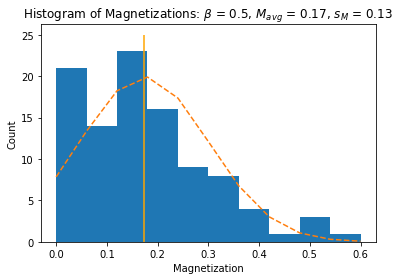The standard deviation sM is visible here as the approximate width of the distribution to either side of the mean. The standard error of the mean σM is much smaller, by a factor of 1/√(Z–1), reflecting its relative stability due to the central limit theory.

## High-Pyrformance Computing

Simulating a large number of variations of a physical model can take a lot of CPU time, and high-performance computing systems provide a lot of CPUs.

### The Amherst College High-Performance Computing System

Larger lattices can take a long time to process in the loop above, and they must then be averaged over a number of different configurations. While the script above should run well in Spyder, it will be very slow overall.

We can therefore turn to the Amherst College High-Performance Computing System, which provides a CPU cluster with 1024 CPU cores on 16 Unix computers, and has Python already installed.

On the CPU cluster we can run a number of calculations, or jobs, on these multiple cores and for longer periods of time.

Cluster jobs are prepared, tested, and submitted on an entry computer or login node, which you can access over the Internet from on-campus or while using a virtual private network (VPN) to appear to be on-campus. The HPC system’s login nodes are accessed via the domain name hpc.amherst.edu, or simply hpc.

General instructions for using the HPC system can be viewed in a web browser at https://hpc.amherst.edu/.

### Procedure 1: Connecting to the HPC System

The HPC system’s computing environment requires the use of text commands rather than a graphical user interface, so you’ll need to open a command-line interpreter, also called a “terminal” or “shell” program, and log in to the login node of the HPC system over the network.

1. Here are complete instructions for this procedure.
2. It’s best to have a dedicated folder/directory for each project, so “make a new directory” in your home folder to contain your script and associated files, e.g.:
3. mkdir ising

4.  Now “change directory” into this new folder, e.g.:
5. cd ising

### Procedure 2: Sharing Files with the HPC System

To submit jobs to the HPC system, you must copy your Python script over to it. You can use file sharing, SCP, or SFTP.

File sharing will probably be more familiar to you, as the network drives appear as if they are local, like your G: drive.

File sharing also allows you to continue to edit within Spyder and retain permissions you’ve set, so it’s recommended.

1. Here are complete instructions for this procedure.
2. The folder username should open, and you should see any subfolders you might have created in Procedure 1, e.g. ising.

You can now copy files into and out of it by dragging them, etc.

Warning: Unix, Windows, and Macs all use different characters to indicate the end of line in a text file; usually they are successfully translated when copying between them, but occasionally they won’t be and error messages may appear in the following steps. This most commonly occurs when transferring from a Windows computer, and if that’s the case you can use the Unix utility dos2unix to ensure a file created on Windows with DOS line-endings has the correct format. Once you’ve converted the file Spyder will respect that end-of-line setting.

### Python Scripts on the HPC System

Once you’ve mapped a network drive to your local computer as in Procedure 2, you can also use the Spyder IDE to read and write files on it.

### Procedure 3: Setting Up Spyder to Use the HPC System

To work with your Python script on the HPC system, it’s simplest to set up Spyder to reference your working folder in hpc.

1. In Spyder, click on the button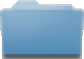Browse a working directory and navigate to the folder to which you connected in Procedure 2, e.g. ising. This folder will remain in the adjacent list of working directories for easy access.
2. To have iPython see this same directory, using one of the following options:
• Click on the IPython button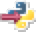Set as current console’s working directory; however this doesn’t work in recent versions of IPython.
• Restart IPython by menuing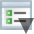Options >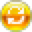Restart kernel. Unfortunately all previous assignments and definitions will be lost, though command history is not.
• Type IPython’s built-in “change directory” command, though you’ll have to type out the full path:
• Macs: %cd /Volumes/username/ising
• Windows: %cd Z:\ising

The % is only necessary if you have already defined a Python variable named cd.

To verify that the directory change worked, type IPython’s built-in “print working directory” command:

%pwd

3. Copy your Python script, e.g. ising.py, to the folder in step 1, from within Spyder by menuing File > Save as….

When running your script on the HPC system, there are a few changes you’ll want to make so that it works more simply with Unix and the HPC system’s job management software.

### Procedure 4: Making a Python Script Self-Executable

To simplify the execution of your script, you can make it run as a “self-executable” Unix application, rather than as a file that’s an argument for the Python interpreter.

1. So that Unix knows which interpreter to use for your script, add the following statement as its first line of code, with no preceding space, and save it:
2. #! /usr/bin/env python3

The first two characters are known as a shebang, and tell Unix to run the script as the next argument of the command that follows.

The Python interpreter’s location can be different for different versions of Python, and can also vary between the HPC system and other Unix computers. In particular, Python 2 is currently the default version on the HPC system, and is located at /usr/bin/python, while Python 3 is located in in the “local binary” folder /usr/local/bin, but it can often be found in other locations. Asking the env program to launch the version in your path is the safest and most general approach.

Because this statement appears as a comment to Python, it will just ignore it when it is handed the script.

3. To get Unix to recognize that your script can be executed directly you must now switch to your command-line program set up in Procedure 1 , make sure you are in the same directory as your script, and “change mode” to include executable status, e.g.:
4. chmod +x ising.py

5. To review the executable status of your script (and other information), you can use the Unix “list -long” command:
6. ls -l

The three groups of permissions here, rwx, r--, and r-- refer to read, write, and execute permissions for you (the user), your group, and all others. It is followed by your username and your group name, and the last-modified date of the file.

7. Now you can run your executable; for security reasons you must explicitly refer to its directory, and since your current directory has the abbreviation . the command will look something like:
8. ./ising.py

Mavg = 0.772
sM = 0.3658740766985
sigmaM = 0.0367717282705

One additional useful capability is to be able to hand your executable one or more arguments that could have different values for different jobs in the HPC system.

In Unix, such command-line arguments are typed following the initial command and separated by spaces, e.g.

./ising.py 1

where 1 might be a specific value of a variable such as beta.

If you import the “system” or sys module into your executable:

import sys

then you will have access to command-line arguments, passed to your executable as a list of strings:

sys.argv

⇒ ['./ising.py', '1']

beta = float(sys.argv)

⇒ 1.0

Note that item 0 in this list is set to the command you used to run the executable.

In general you will want to test to make sure that the expected number of arguments are provided, and either set a default value for missing arguments or exit the program with an error message (another function provided by the sys module):

if len(sys.argv) < 2 :
sys.exit('Usage: ' + sys.argv + ' beta')
else:
beta = float(sys.argv)

Then when running the executable without arguments:

./ising.py

⇒ Usage: ./ising.py beta

If you need to engage in more complicated argument-list processing, especially if you want to include options like -t (e.g. to allow for keyword rather than positional arguments), take a look at the getopt module.

One other important change for your executable is to format its output to make it easier to analyze large quantities of data; most simply, you can write comma-separated values so that the results of different jobs can all be concatenated together into a table:

print('L,Z,beta,Mavg,sM,sigmaM')
print('%d,%d,%0.2f,%0.3f,%0.3f,%0.3f' % (L,Z,beta,Mavg,sM,sigmaM))

L,Z,beta,Mavg,sM,sigmaM
10,100,10.00,0.77,0.37,0.038

### The Slurm Job-Management System

The Slurm job-management software provides an efficient means to control a project running on a large number of computers.

A set of related jobs are submitted as a cluster of jobs that are automatically distributed over any available computer cores.

By design CPU time is shared with other researchers; the general behavior is that newer clusters are given priority, and the longer they run the lower their priority becomes.

Along with your executable program, Slurm will need a batch file for each job cluster, which provides details on how to run each job, including any command-line arguments that might vary from job to job.

The basic structure of a batch file is a series of commands, one per line, usually key=value pairs that describe either global job properties or individual job properties, for example:

## Global job properties
universe     = vanilla
requirements = (((Arch=="INTEL") || (Arch=="x86_64")) && (OpSys=="LINUX"))
priority     = 5

## Job properties
arguments = 0.1
queue

## Job properties
arguments = 0.2
queue
...

As with Python, a # introduces a comment.

The keyword universe describes possible modes of running your executable; when the jobs are independent of each other (don’t need to pass information amongst themselves), the proper value is vanilla. There are other possiblities for more specialized applications, e.g. for code compiled with parallel-processing libraries.

The keyword notification determines whether an e-mail will be sent for certain situations:

• complete (the default): upon job completion, whether properly or due to errors
• error: only when job errors occur
• never

The complete option is not generally recommended, because it can generate hundreds of e-mails, one for each job, which look like:

This is an automated email from the Slurm system
on machine "csc2-node01.amherst.edu". Do not reply.

Slurm job 150601.0
has exited normally with status 0
....

If you set notification to something other than never, you should include your e-mail address using the notify_user keyword.

The keyword executable is the location of the application that Slurm is going to run.

The keyword requirements describes various node characteristics necessary for your application, in particular the hardware architecture (Arch) and operating system (OpSys), together known as the node platform. Since Slurm runs on heterogeneous equipment, but many applications cannot, this keyword is important to set properly, especially since the default platform characteristics are the same as the login node. The requirements can both be limited with an AND statement (&&) or expanded with an OR statement (||). For the Amherst College HPC system the value combination above describes all of its platforms, which all run the Linux operating system, but which are either AMD or Intel CPUs — and therefore run the same compiled binary code.

The keyword priority is any integer and is a relative priority for your own jobs — a lower priority, such as 0 (the default), will be run only after your other jobs with higher priority have run. This doesn’t effect job priority relative to other users, which is set by Slurm using its own algorithm.

The keyword arguments is a list of text values that will be handed to your application, e.g. ising.py 0.1.

The keyword initialdir is the working directory where each instance of the application will run and where its varying input and output files are or will be located. Keeping them in the same directory allows the app to read and write with local references no matter which instance it is. Common input files can be referenced either with full paths or with relative references, e.g. ../.. to refer to the base directory.

The keywords output, error, and log describe files to store, respectively, standard output from your application (what would appear as output if you ran it directly), any error messages that occur, and Slurm’s log of running the job providing information similar to the e-mail above.

The keyword queue tells Slurm to submit one job with the previous set of characteristics. You can then change a few of them for the next job, and queue that. The variant queue n submits n jobs all with the same characteristics, useful when other parameters are created randomly by the program.

There are many more commands that might occasionally be useful and that are described in the Slurm documentation.

Once you’ve created your batch file, use Slurm_submit to send your project to the Slurm job queue.

### Example: Creating a Slurm Batch File Using Python

A Slurm batch file is required to run a project on the HPC system, to describe the characteristics of each job in the project cluster.

The batch file can be very long and the arguments and files will vary from job to job, so it’s convenient to create it from a program, using almost any language, but the example here will be Python (of course). This is especially useful when job arguments can be generated algorithmically.

For the Monte Python simulation above, an example Slurm batch file generator “ising.sb” is downloadable here, and is detailed below:

1. As with your application, you’ll need to reference the full path to the location of the Python intrepreter with a shebang on the first line of the generator:
2. #! /usr/bin/env python3

3. Because the batch file references locations in the file directory, the generator can make use of the “operating system” module os to obtains its own “current working directory” using os.getcwd(), so that it can create the batch file and the results directories in the same place:
import os, math

# Create file references for this project:
projectName = 'ising'
baseDirectory = os.getcwd()
executable = baseDirectory + '/' + projectName + '.py'
commandFile = baseDirectory + '/' + projectName + '.sb'

The generator first writes out global job parameters into the batch file:

# Write the global parameters needed for all jobs into the batch file:
commands = open(commandFile, 'w')
commands.writelines(['## Global job properties\n',
'universe =     vanilla\n',
'notify_user  = username@amherst.edu\n',	'getenv =       true\n',
'executable =   ' + executable + '\n',
'requirements = (((Arch=="INTEL") || (Arch=="x86_64")) && (OpSys=="LINUX"))\n',
'priority =     5\n'])

These are:

• a “vanilla universe” (meaning no communication between the different jobs);
• notification on error, but not upon job completion (you might want to change this to complete for a long-running job);
• provide the executable with your environment variables (required for some programs, also required to make #! /usr/bin/env python3 work).
• the locations of the initial directory and the project executable;
• hardware requirements (here redundant since all of the HPC system CPUs match);
• the project running priority, which you can use to prioritize your own projects (lower is better, but your priority relative to others is beyond your control):

The results directory is created using more functions from the os module, with rwx permissions for yourself but not your group (---) or others (---), which can be written as a binary number 0b 111 000 000 or as an octal number 0o700 (the default is world-writable 0o777, which may be necessary for some Slurm configurations):

# Create the results directory and limit accessibility to yourself with
# the permission rwx --- --- (0o700):
baseResultsDirectory = baseDirectory + '/results'
if not os.path.exists(baseResultsDirectory) :
os.mkdir(baseResultsDirectory, 0o700)

The job arguments are generated with a simple algorithm for the values of beta to be used, which are placed in a list and counted:

# Job arguments, here [0.1, 0.2, 0.3, …, 1.3, 1.4, 1.5]:
betaValues = [(x+1)/10 for x in range(15)]
jobsCount = len(betaValues)

Each job will have its own results directory within the main results directory, and giving them a uniform-length filename will allow them to be sorted in numerical order:

# Create a format string that will produce results directory names that
# have a standard length for sorting purposes, e.g. 'results/%02d'
#  => 'results/00', ..., 'results/09', 'results/10', ..., 'results/99'resultsDirectoryFormat = baseResultsDirectory + '/%0' + \
str(math.floor(math.log10(jobsCount - 1)) - 1) + 'd'

Now loop through the jobs and create their individual results directories by applying the string format operator:

# Loop through the parameters to be passed for each job:
for job in range(jobsCount) :

# Create the results directory for this specific job.
resultsDirectory = resultsDirectoryFormat % job
if not os.path.exists(resultsDirectory) :
os.mkdir(resultsDirectory, 0o700)

In this loop the parameters for each job are also written into the batch file:

• the argument(s) for each job are the corresponding value of beta;
• the output file has the data your executable writes;
• the error file describes issues that occur as the executable runs, e.g. due to Python syntax errors;
• the log file has more general details about running the job, and can tell you about non-Python issues such as file permission issues:
    # Write the arguments for this job.
commands.writelines(['\n## Job properties\n',
'arguments = ' + str(betaValues[job]) + '\n',
'initialdir =   ' + resultsDirectory + '\n',
'output = ' + resultsDirectory + '/out\n',
'error = ' + resultsDirectory + '/err\n',
'log = ' + resultsDirectory + '/log\n',
'queue\n'])

Finally, close the batch file:

commands.close()

The batch file generator should be saved on the HPC system in the same location as your project executable.

As with your executable, the batch file generator should be provided with executable permissions:

chmod +x ising.sb


The batch file generator can then be handed to sbatch to run:

./ising.sb


And you should also verify the presence of the results directories:

ls results
⇒
00  01  02  03  04  05  06  07  08  09  10  11  12  13  14  15

### Procedure 6: Running a Project on the HPC System

Once you have an application and a Slurm batch file describing how to run it, such as described above, there are just a few commands to know to get it running on the HPC system:

1. To submit a project batch file to Slurm:
2. sbatch ising.sb
⇒
Submitting job(s)Logging submit event(s)..........15 job(s) submitted to job id 149415.

Note the job ID 149415, which allows you to reference this job and its set of tasks.

3. To monitor your Slurm jobs:
4. squeue --me
⇒
-- Submitter: csc2-node01.amherst.edu : <148.85.78.21:46911>
ID      OWNER        SUBMITTED     RUN_TIME ST PRI SIZE CMD
149415.0   username   12/18 16:56   0+00:03:34 R  5   9.8  ising.py 0.1
149415.1   username   12/18 16:56   0+00:03:34 R  5   9.8  ising.py 0.2

149415.14  username   12/18 16:56   0+00:03:34 R  5   9.8  ising.py 1.4
149415.15  username   12/18 16:56   0+00:03:34 R  5   9.8  ising.py 1.5

Total for query: 15 jobs; 0 completed, 0 removed, 0 idle, 15 running, 0 held, 0 suspended
Total for username: 15 jobs; 0 completed, 0 removed, 0 idle, 15 running, 0 held, 0 suspended
Total for all users: 15 jobs; 0 completed, 0 removed, 0 idle, 15 running, 0 held, 0 suspended

With the option -nobatch, the individual jobs are listed line-by-line and identified as <clusterID>.<processID>, e.g. 149415.0, 149415.1, etc.

The actual command that Slurm is running is shown at the end, and you should verify that it’s what you expect.

The status of each job is listed in the ST column, with R for running, I for idle, and H for on hold. Idle jobs are waiting for their turn to run, and will appear most commonly for long-running jobs. Held jobs are generally put in that state manually with the command Slurm_hold, but can sometimes end up there due to errors in the job.

5. To remove Slurm jobs, particularly important if the results aren’t what you expect, your jobs are unexpectedly on hold, etc.:
6. scancel 149415 149416.9

The command arguments are a list of cluster IDs and/or cluster.process IDs, or your user ID to remove all of your jobs.

7. If your job does not complete, or has unexpected results, check the err and log files in each result directory for messages that describe any issued with your code or the file itself.

There are many more commands that might occasionally be useful and which are described in the Slurm documentation.

### Procedure 7: Processing Your Results

The results of your cluster job are now stored in the directories results/00/out, results/01/out, ….

1. To collect your output together in one file:
2. cat results/*/out > ising.csv

The wild card character * will match any of the job directories 0, 1, …, and the command cat concatenates them together. The standard output redirect character > takes the result, which would otherwise be printed, and places it in the new file named ising.csv in your current directory.

3. The content of ising.csv is in the format known as comma-separated values, which can be easily read with Excel and other programs:
4. cat ising.csv
⇒
L,Z,beta,Mavg,sM,sigmaM
10,100,0.10,0.095,0.075,0.008
L,Z,beta,Mavg,sM,sigmaM
10,100,0.20,0.090,0.066,0.007
....
L,Z,beta,Mavg,sM,sigmaM
10,100,1.40,1.001,0.017,0.002
L,Z,beta,Mavg,sM,sigmaM
10,100,1.50,1.005,0.014,0.001
5. The header lines, included with each file in case you need to look at them individually, can be removed by sorting with Excel, selecting them, and deleting them.

More esoterically the extra lines can be removed with the Unix stream editor sed:
6. cat results/*/out | sed -e 1n -e /L/d > ising.csv

The pipe character | redirects the standard output of the cat command into the standard input of the next command, sed (a combination known as a pipeline).

The sed command applies two edits with the option -e, the first 1n to write the first line and move on to the next, the second /L/d to match any other line that includes the text L and delete it:

cat ising.csv
⇒
L,Z,beta,Mavg,sM,sigmaM
10,100,0.10,0.085,0.075,0.008
10,100,0.20,0.090,0.066,0.007
....
10,100,1.40,1.001,0.017,0.00210,100,1.50,1.005,0.014,0.001

Unix has a wealth of such commands to process the content of files, you can find a list of them on Wikipedia.

7. You can import this file using the general techniques described previously, but here we’ll use the numpy method loadtxt, which simplifies the process by recognizing the file’s tabular structure (and lets us easily skip the header row to get just numeric input):
8. ising = np.loadtxt('ising.csv', delimiter=',', skiprows = 1)

ising
⇒
array([[1.000e+01, 1.000e+02, 1.000e-01, 8.500e-02, 7.500e-02, 8.000e-03],
[1.000e+01, 1.000e+02, 2.000e-01, 9.000e-02, 6.600e-02, 7.000e-03],
....
[1.000e+01, 1.000e+02, 1.400e+00, 1.001e+00, 1.700e-02, 2.000e-03],
[1.000e+01, 1.000e+02, 1.500e+00, 1.005e+00, 1.400e-02, 1.000e-03]])
9.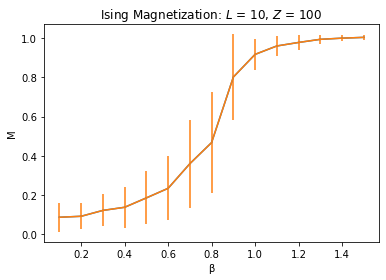And then you can plot this data, this time adding error bars:
10. import matplotlib.pyplot as plt
plt.plot(ising[:,2], ising[:,3])
plt.errorbar(ising[:,2], ising[:,3], ising[:,4])
plt.xlabel('β')
plt.ylabel('M')
plt.title(r'Ising Magnetization: $L$ = ' + str(L) + ', $Z$ = ' + str(Z))
plt.show()
plt.close()


### Example: Running Jobs with Self-Generated Data

Some jobs will generate all of their own data, typically from some statistical distribution, and so don’t need inputs via the command line. They do need to write their outputs in different files, and Slurm provides a means for that. Consider the following batch file “sample.sb”, which you can download here:

#! /bin/sh
#SBATCH --output=ising-%j.out
#SBATCH --error=ising-%j.err

# Check for the results directory, and if it isn't present, create it:
if [[ ! -d 'results' ]]; then mkdir 'results'; fi

# Hand the python script to Slurm to run, each task on its own cpu, and wait for them to complete:
srun --overlap -o results/ising-%j-%2t.out -e results/ising-%j-%2t.err ./ising.py
wait


This is written for an R script, rather than Python, but hopefully the procedure is still clear.

### Problem 6: The Full Monte

Using the HPC system, repeat Procedure 7 over larger arrays L and more ensembles (e.g. Z = 1000), by generalizing the command-line input of your executable to allow run-time choices of these values. Calculate in each case the magnetization (using the approximation for large Z):

M⟩ = (1/Z) ΣZ M

the average square magnetization:

M2⟩ = (1/Z) ΣZ M 2

and then the standard deviation of the mean value of M:

sM = sqrt(M2⟩ – ⟨M2)

Plot the results for different values of L on one graph; what happens as L gets larger?

### Scientific Programming with Python:Simulating a Magnet

Top of Page  | Using IT DocumentationIT Home  |  IT Site Map  |  Search IT